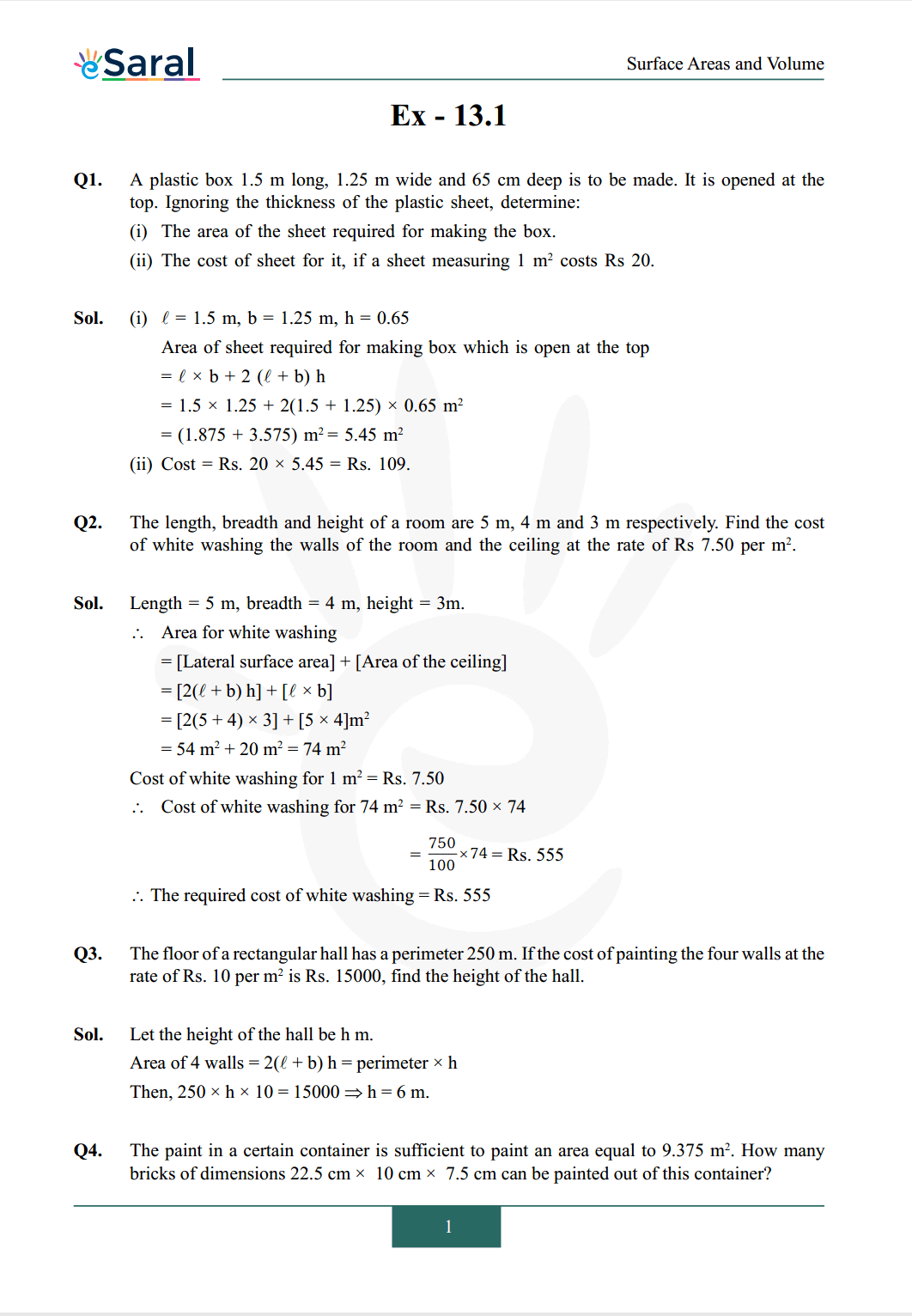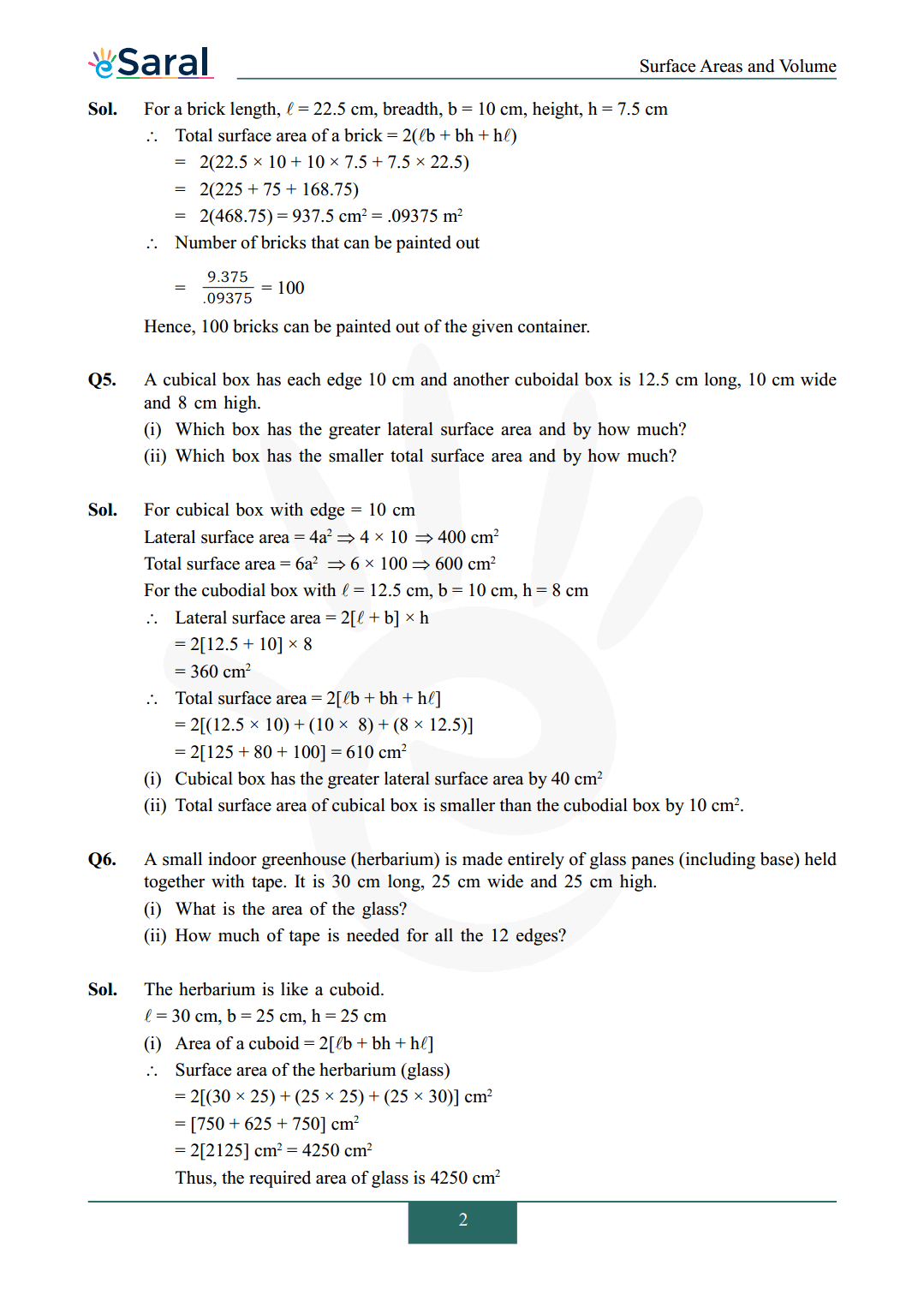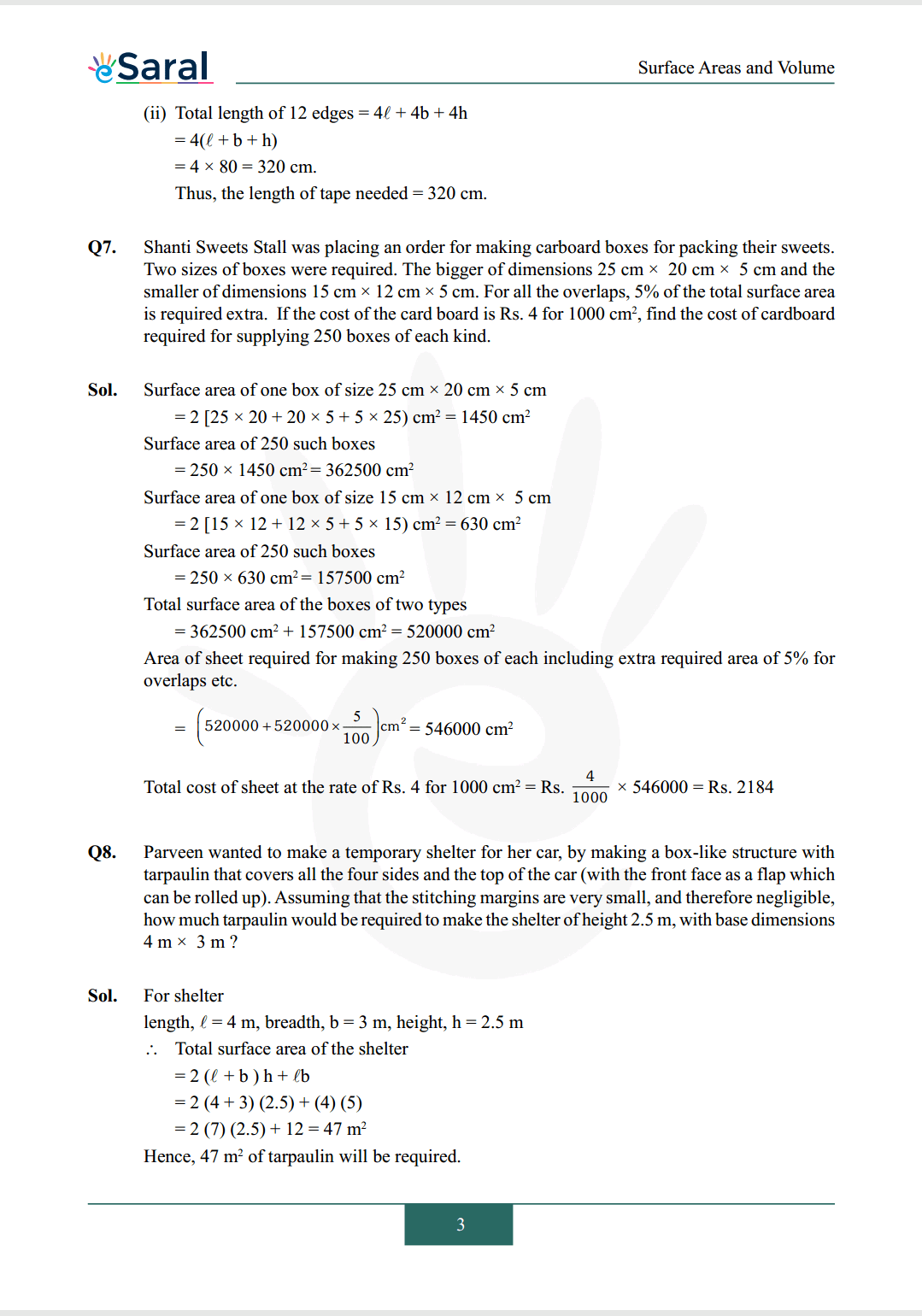# NCERT Solutions for Class 9 Maths chapter 13 Exercise 13.1 - Surface Areas and VolumesHey, are you a class 9 Student and Looking for Ways to Download NCERT Solutions for Class 9 Maths chapter 13 Exercise 13.1? If Yes then you are at the right place.

Here we have listed Class 9 maths chapter 13 exercise 13.1 solutions in PDF that is prepared by Kota’s top IITian’s Faculties by keeping Simplicity in mind.

If you want to score high in your class 9 Maths Exam then it is very important for you to have a good knowledge of all the important topics, so to learn and practice those topics you can use eSaral NCERT Solutions.

So, without wasting more time Let’s start.

### Download The PDF of NCERT Solutions for Class 9 Maths chapter 13 Exercise 13.1 "Surface Areas and Volumes"#### All Questions of Chapter 13 Exercise 13.1

Once you complete the chapter 13 then you can revise Ex. 13.1 by solving following questions

Q1. A plastic box $1.5 \mathrm{~m}$ long, $1.25 \mathrm{~m}$ wide and $65 \mathrm{~cm}$ deep is to be made. It is opened at the top. Ignoring the thickness of the plastic sheet, determine:
(i) The area of the sheet required for making the box.
(ii) The cost of sheet for it, if a sheet measuring $1 \mathrm{~m}^{2}$ costs Rs 20.

Q2. The length, breadth and height of a room are $5 \mathrm{~m}, 4 \mathrm{~m}$ and $3 \mathrm{~m}$ respectively. Find the cost of white washing the walls of the room and the ceiling at the rate of Rs $7.50$ per $\mathrm{m}^{2}$.

Q3. The floor of a rectangular hall has a perimeter $250 \mathrm{~m}$. If the cost of painting the four walls at the rate of Rs. 10 per $\mathrm{m}^{2}$ is Rs. 15000, find the height of the hall.

Q4. The paint in a certain container is sufficient to paint an area equal to $9.375 \mathrm{~m}^{2}$. How many bricks of dimensions $22.5 \mathrm{~cm} \times 10 \mathrm{~cm} \times 7.5 \mathrm{~cm}$ can be painted out of this container?

Q5. A cubical box has each edge $10 \mathrm{~cm}$ and another cuboidal box is $12.5 \mathrm{~cm}$ long, $10 \mathrm{~cm}$ wide and $8 \mathrm{~cm}$ high.
(i) Which box has the greater lateral surface area and by how much?
(ii) Which box has the smaller total surface area and by how much?

Q6. A small indoor greenhouse (herbarium) is made entirely of glass panes (including base) held together with tape. It is $30 \mathrm{~cm}$ long, $25 \mathrm{~cm}$ wide and $25 \mathrm{~cm}$ high.
(i) What is the area of the glass?
(ii) How much of tape is needed for all the 12 edges?

Q7. Shanti Sweets Stall was placing an order for making carboard boxes for packing their sweets. Two sizes of boxes were required. The bigger of dimensions $25 \mathrm{~cm} \times 20 \mathrm{~cm} \times 5 \mathrm{~cm}$ and the smaller of dimensions $15 \mathrm{~cm} \times 12 \mathrm{~cm} \times 5 \mathrm{~cm}$. For all the overlaps, $5 \%$ of the total surface area is required extra. If the cost of the card board is Rs. 4 for $1000 \mathrm{~cm}^{2}$, find the cost of cardboard required for supplying 250 boxes of each kind.

Q8. Parveen wanted to make a temporary shelter for her car, by making a box-like structure with tarpaulin that covers all the four sides and the top of the car (with the front face as a flap which can be rolled up). Assuming that the stitching margins are very small, and therefore negligible, how much tarpaulin would be required to make the shelter of height $2.5 \mathrm{~m}$, with base dimensions $4 \mathrm{~m} \times 3 \mathrm{~m} ?$